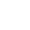# jMEF

## A Java library to create, process and manage mixtures of exponential families

View ongithub

# What are exponential families?

An exponential family is a generic set of probability distributions that admit the following canonical distribution:

$$\normalsize p_F(\Theta) = \exp(\langle \Theta, t(x)\rangle - F(\Theta) + k(x) )$$

Exponential families are characterized by the log normalizer function F, and include the following well-known distributions: Gaussian (generic, isotropic Gaussian, diagonal Gaussian, rectified Gaussian or Wald distributions, lognormal), Poisson, Bernoulli, binomial, multinomial, Laplacian, Gamma (incl. chi-squared), Beta, exponential, Wishart, Dirichlet, Rayleigh, probability simplex, negative binomial distribution, Weibull, von Mises, Pareto distributions, skew logistic, etc. All corresponding formula of the canonical decomposition are given in the documentation.

Mixtures of exponential families provide a generic framework for handling Gaussian mixture models (GMMs also called MoGs for mixture of Gaussians), mixture of Poisson distributions, and Laplacian mixture models as well.

# What is jMEF?

jMEF is a Java cross-platform library developped by Vincent Garcia and Frank Nielsen. jMEF allows one to:

• create and manage mixture of exponential families (MEF for short),
• estimate the parameters of a MEF using Bregman soft clustering (equivalent by duality to the Expectation-Maximization algorithm),
• simplify MEFs using Bregman hard clustering (k-means algorithm in natural parameter space),
• define a hierachical MEF using Bregman hierarchical clustering,
• automatically retrieve the optimal number of components in the mixture using the hierarchical MEF structure.

# Related bibliography

1. Vincent Garcia, Frank Nielsen, and Richard Nock
Levels of details for Gaussian mixture models
In Proceedings of the Asian Conference on Computer Vision, Xi'an, China, September 2009
2. Frank Nielsen, and Vincent Garcia
Statistical exponential families: A digest with flash cards
arXiV, http://arxiv.org/abs/0911.4863, November 2009
3. Frank Nielsen, Vincent Garcia, and Richard Nock
Simplifying Gaussian mixture models via entropic quantization
In Proceedings of the European Signal Processing Conference (EUSIPCO), Glasgow, Scotland, August 2009
4. Frank Nielsen and Richard Nock
Sided and symmetrized Bregman centroids
IEEE Transactions on Information Theory, 2009, 55, 2048-2059
5. Frank Nielsen, Jean-Daniel Boissonnat and Richard Nock
On Bregman Voronoi diagrams
ACM-SIAM Symposium on Data Mining, 2007, 746-755
6. A. Banerjee, S. Merugu, I. Dhillon, and J. Ghosh
Clustering with Bregman divergences
Journal of Machine Learning Research, 2005, 6, 234-245